Top four LaTeX mistakes

Here are four of the most common typesetting errors I see in books and articles created with LaTeX.

1) Quotes

Quotation marks in LaTeX files begin with two back ticks, , and end with two single quotes, ''.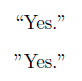The first “Yes” was written as

Yes.''

in LaTeX while the one with the backward opening quote was written as

"Yes."

2) Differentials

Differentials, most commonly the dx at the end of an integer, should have a little space separating them from other elements. The “dx” is a unit and so it needs a little space to keep from looking like the product of “d” and “x.” You can do this in LaTeX by inserting \, before and between differentials.The first integral was written as

 \int_0^1 f(x) \, dx

while the second forgot the , and was written as

 \int_0^1 f(x)  dx

The need for a little extra space around differentials becomes more obvious in multiple integrals.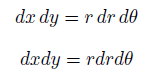The first was written as

dx \, dy = r \, dr \, d\theta

while the second was written as

dx  dy = r  dr  d\theta

3) Multi-letter function names

The LaTeX commands for typesetting functions like sin, cos, log, max, etc. begin with a backslash. The command log keeps “log,” for example, from looking like the product of variables “l”, “o”, and “g.”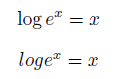The first example above was written as

\log e^x = x

and the second as

log e^x = x

The double angle identity for sine is readable when properly typeset and a jumbled mess when the necessary backslashes are left out.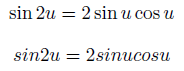The first example was written

\sin 2u = 2 \sin u \cos u

and the second as

sin 2u = 2 sin u cos u

4) Failure to use math mode

LaTeX uses math mode to distinguish variables from ordinary letters. Variables are typeset in math italic, a special style that is not the same as ordinary italic prose.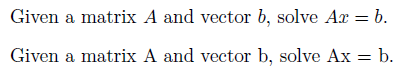The first sentence was written as

Given a matrix $A$ and vector $b$, solve $Ax = b$.

and the second as

Given a matrix A and vector b, solve Ax = b.

Related posts: